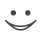# Get these flashcards, study & pass exams. For free! Even on iPhone/Android!

## Metric Units (16 Cards)

Larger unit to a smaller unit?
kilometer = meter
X 1000

ie., 5 km X 1000 = 5,000m

Smaller unit to a larger unit?
Centimeters = meters
1/100

ie., 500 cm /100 = 5m
What are the hecto measurements?
Hectometer

Hectogram

Hectoliter

( X 1000 )

What are the deca measurements?
Decameter

Decagram

Decaliter

( X 10 )

What are the base measurements?
Meter

Gram

Liter
What are the deci measurements?
Decimeter

Decigram

Deciliter

( / 10 )
What are the centi measurements?
Centimeter

Centigram

Centiliter

( / 1000 )
What are the milli measurements?
Millimeter

Milligram

Milliliter

( / 1000)
What are the kilo measurements?
Kilometer

Kilogram

Kiloiter

( X 1000 )
Kilometer =
1000 meters
Hectometer =
100 meters
Decameter =
10 meters
Decimeter =
1/10 meter
Centimeter =
1/100 meter
Millimeter =
1/1000 meterFlashcard set info:
Author: Andrew R
Main topic: Mathematics
Topic: Metric units
Published: 23.02.2010
Tags: math, leter

Card tags:
All cards (16)
no tagsReport abuse